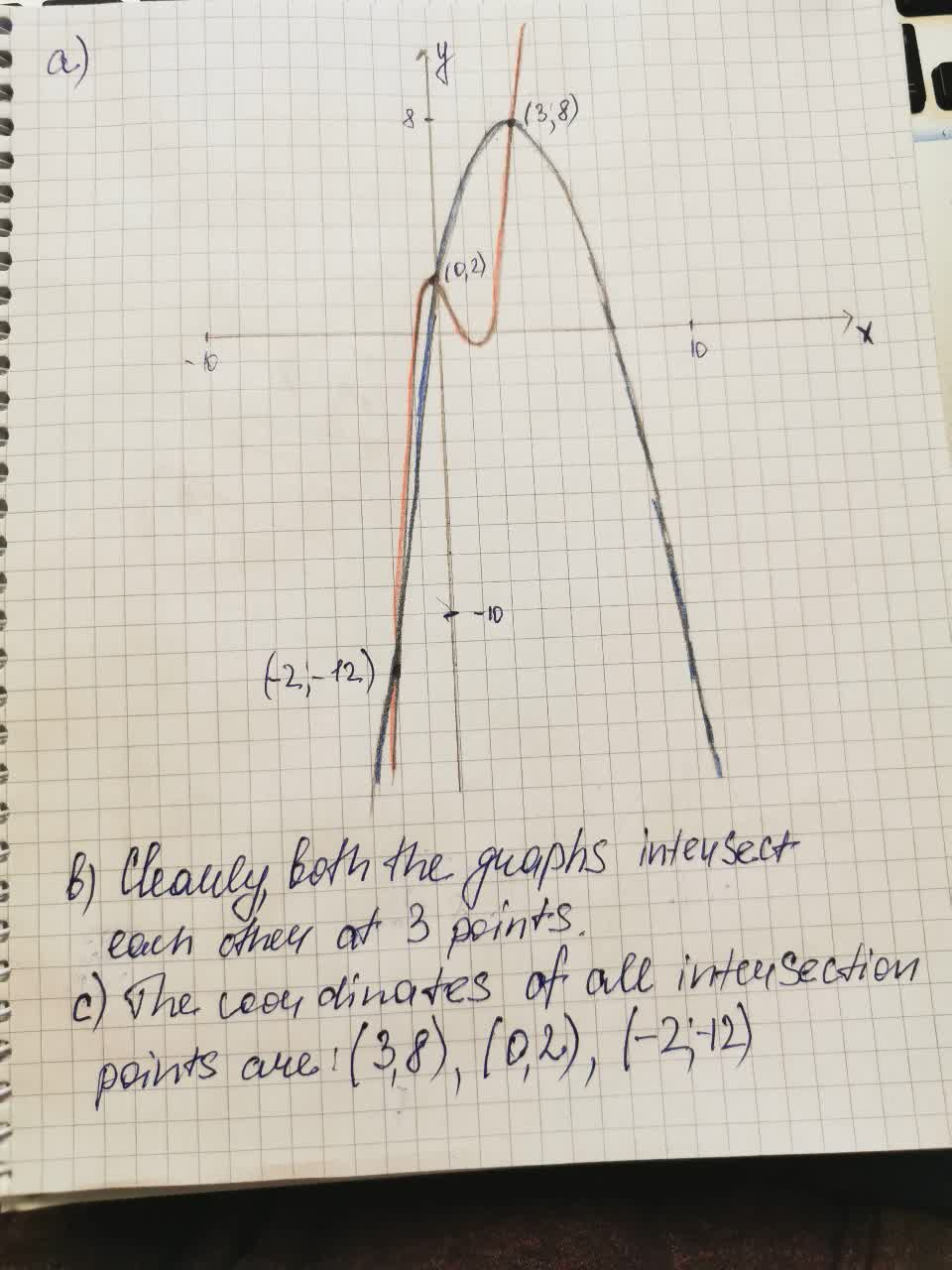# y=x^3-2x^2-x+2 and y=-x^2+5x+2 To determine:a) Graph of both functions b) At howCoormaBak9 2021-08-11 Answered
$y={x}^{3}-2{x}^{2}-x+2$
and $y=-{x}^{2}+5x+2$
To determine:a) Graph of both functions
b) At how many points do the both graphs appear to intersect.
c) Find coordinates of all intersection points
You can still ask an expert for help

• Questions are typically answered in as fast as 30 minutes

Solve your problem for the price of one coffee

• Math expert for every subject
• Pay only if we can solve itcasincal

To determine: